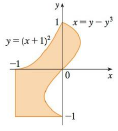Chapter 15.2, Problem 58E

Chapter
Section
Textbook Problem

Express D as a union of regions of type I or type II and evaluate the integral.58. ∬ D y   d ATo determine

To express: The region D as a union of regions of type 1 or type 2 and find the value of given double integral.

Explanation

Region of type 1:

A plane region D is said to be of type 1 if it lies between two continuous functions of x.

That is, D={(x,y)|axb,g1(x)yg2(x)} ,where g1(x) and g2(x) are the continuous functions of x.

Region of type 2:

A plane region D is said to be of type 2 if it lies between two continuous functions of y.

That is, D={(x,y)|ayb,h1(y)xh2(y)} ,where h1(y) and h2(y) are the continuous functions of y.

Calculation:

The given region D as a type 2 region is split into two parts. In first part, x varies from 1 to yy3 and y varies from 1 to 0. In second part, x varies from y1 to yy3 and y varies from 0 to 1. So, the value of the double integral is,

Df(x,y)dA=101yy3ydxdy+01y1yy3ydxdy

First, compute the integral with respect to x.

101yy3ydxdy+01y1yy3ydxdy=10[xy]1yy3dy+01[xy]y1yy3dy

Apply the limit value for x,

101yy3ydxdy+01y1yy3ydxdy=1

Still sussing out bartleby?

Check out a sample textbook solution.

See a sample solution

The Solution to Your Study Problems

Bartleby provides explanations to thousands of textbook problems written by our experts, many with advanced degrees!

Get Started

Find more solutions based on key concepts